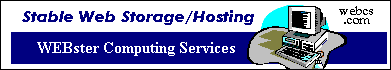Logic Chapter Two
Next Chapter

OPERATORS

An operator is a way of making statements into other statements (called "complex" statements) that mean more or less different things. There's a bunch of operators out there, but to keep things simple I'm going to start with just three operators. They are known as "NOT," "AND," and "OR."

NOT

The first operator is "~," the "tilde" (or "snake," or you can call it "the not-sign" or just "NOT."), and it reverses the truth-value of whatever statement it's attached to. Here's how it works.

Adding a tilde to "Bb" we get "~Bb," which means "Bill Clinton is not a bear." (The thing the operator operates on is called a "term," which means that "Bb" is the term of "~Bb.")

Adding another tilde to "~Bb" we get "~ ~Bb," which means "Bill Clinton is not not a bear." (Now this means the same thing as Bb, but they're not the same statement. Trust me.)

Adding yet another tilde we... oh, you can figure it out. You can have as many tildes in a statement as you like, make it an enormous conga line of snakes, but every one you add reverses the truth value of the statement you add it to. (Remember that "P" and "Q" stand for any statement)

If P is true, then ~P is false, but ~ ~P is true, and ~ ~ ~P is false!

But if Q is false, then ~Q is true, but ~ ~Q is false, and ~ ~ ~Q is (you guessed it) true!

For practice, work out the values of the negations of our four original statements.~Ba = ? ~Bb = ? ~Ca = ? ~Cb = ?

TRUTH TABLES

You may have noticed that worlds are basically defined in terms of what is or isn't true in a particular situation. Sometimes, we will want to specify several different worlds all at once. For this, we use truth tables.

Here is a truth table that covers all the possible values of Ba and Cb.If I was to represent all these possible values in Venn diagrams, or "world pictures," it would look like this:Holy crap! That's a lot of worlds! And damned confusing too! If you look closely, you'll see that some of these worlds make Ba and Cb both true, some make Ba true and Cb false, (which is the same as making Ba true and ~Cb true), some make Ba false and Cb true (~Ba and Cb both true), and finally, some make Ba and Cb both false, (or ~Ba and ~Cb both true). Since all I care about right now is the values of Ba and Cb, I'm going to forget about all these worlds and just go with the truth table.Look at the "T"s and "F"s. As you may guesss, "T" stands for "true" and "F" stands for "false." Each row in a truth table stands for a set of worlds in which the statements in question have the values indicated by the relevant letters. Thus in row 1, Ba and Cb are both true, row 2 Ba is true and Cb is false, in row 3 Ba is false and Cb is true and in row 4, Ba and Cb are both false. A lot easier to keep track of than a bunch of world pictures, huh? (From now on I'm going to use the word "world" to mean both "world" and "set of worlds." Basically, this means a line on a truth table will be called a "world." I hope this doesn't confuse anyone.)

But we don't have to stick to any particular statements. We can do truth tables for any arbitrarily chosen sentences. Here's one for any two different statements, which I will represent by the sentence letters "P" and "Q"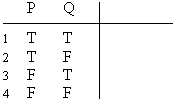Look at the "T"s and "F"s. As before, "T" stands for "true" and "F" stands for "false," and each row in a truth table stands for a world in which the statements in question have the values indicated by the relevant letters. Thus in row 1, P and Q are both true, row 2 P is true and Q is false, in row 3 P is false and Q is true and in row 4, P and Q are both false.

Here's the truth table for any statements that happen to get modified by repeated additions of the "not" operator.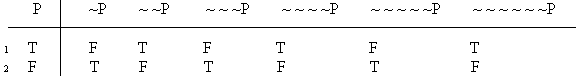Notice that the "F"s and "T"s are always under the first tilde. That's because that tilde is the main operator of that particular statement. The main operator is the last one you think about when evaluating a statement, and it's what makes a statement the kind of statement it is. A statement whose main operator is a tilde is called a "negation."

AND

The next operator is "^," the "carat" (or just "AND") and it joins two other statements (called "terms") together to make a new statement that is true only when both of the joined statements are also true, and false when either or both of them are false. Here's its truth table. A statement whose main operator is a carat is called a "conjunction," and it's terms are called "conjuncts."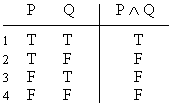Notice that there's only one world in which "P ^ Q" is true. And that world is... ?

Adding a carat to "Bb" we get " ^ Bb," which doesn't mean anything. But if we add "Ca" to that we get "Ca  ^ Bb," which does mean something. It means "King Arthur is cat and Bill Clinton is a bear." That's a statement that's only true if both parts of it are true. If Bill Clinton isn't a bear, then the whole statement "Ca ^ Bb" is false, whether King Arthur is cat or not. And if King Arthur isn't a cat, well...

For practice, work out the values of these conjunctions in these worlds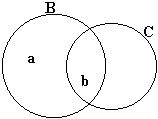Ba ^ Ca Bb ^ Ca Ba ^ Bb Bb ^ Bb Ca ^ Cb Bb ^ Ba Ba ^ Cb Bb ^ Cb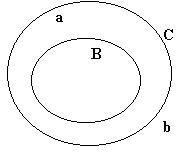Ba ^ Ca Bb ^ Ca Ba ^ Bb Bb ^ Bb Ca ^ Cb Bb ^ Ba Ba ^ Cb Bb ^ CbBa ^ Ca Bb ^ Ca Ba ^ Bb Bb ^ Bb Ca ^ Cb Bb ^ Ba Ba ^ Cb Bb ^ Cb

OR

The next operator is " v," the "wedge" (or just "OR") and it joins two other statements together to make a new statement that is false only when both of the joined statements are also false, and true when either or both of them are true. Here's its truth table. A statement whose main operator is a wedge is called a "disjunction," and its terms are called "disjuncts."Notice that there's only one world in which "P v Q" is false. And that world is... ?

While a conjuction will only be true if both of its conjuncts were true, a disjunction will be true if either of its disjuncts are true. (Here "either" means one, the other, or both.) Thus the following worlds all make the statement "Ca v Ba" true.But none of them make "Ca  ^ Ba" true. That's right, "Ca  v Ba" is true and "Ca  ^ Ba" is false in each of these worlds.
(But remember, "P v Q" is true whenever "P ^ Q" is true)

For practice, work out the values of these disjunctions in these worldsBa v Ca Bb v Ca Ba v Bb Bb v Bb Ca v Cb Bb v Ba Ba v Cb Bb v CbBa v Ca Bb v Ca Ba v Bb Bb v Bb Ca v Cb Bb v Ba Ba v Cb Bb v CbBa v Ca Bb v Ca Ba v Bb Bb v Bb Ca v Cb Bb v Ba Ba v Cb Bb v Cb

Remember that disjunctions (like P v Q) are true just so long as one of their disjuncts are true. So the following sentences are all true:

Some grass is green or all elephants are made of tofu.
Ru Paul is president or cars can run on gasolene
Smoking is bad for you or celery can grow in your ears.

They're true because they're disjunctions and, in each one, at least one of the disjuncts is true. Now, you might think that I'm cheating, because in each one I took a true statement and added something silly. Well, yes, that's what I did. And you can do it too. (Practice on your own for a bit. I'll wait.)

Possible Quiz Questions.

1. Draw a picture in which Fa is true.

2. Draw a picture in which Gb is false.

3. Draw a picture in which ~Hc is true.

4. Draw a picture in which ~Id is false.

5. Draw a picture in which Je ^ Kf is true.

6. Draw a picture in which Lg ^ Mh is false.

7. Draw a picture in which Ni v Fa is true.

8. Draw a picture in which Gb v Hc is false.

9. Draw a picture in which Id is true and Je is false.

10. Draw a picture in which Kf v Lg is true and Lg is false. (Remember that g can't be in two places.)

11. Draw a picture in which Mh is true and Mh ^ Ni is false. (Remember that h can't be in two places.)

12. Draw a picture in which Fi v Gi is true and Gi is false. (Remember that i can't be in two places.)

13. Draw a picture in which Ha is true and Ha ^ Ia is false. (Remember that a can't be in two places.)

14. Is it possible to draw a picture in which Ai ^ Bj is true and Ai is false. (Remember i can't be two places.)

15. Is it possible to draw a picture in which Ck is true and Ck v Dl is false. (Remember k can't be two places.)

16. Is it possible to draw a picture in which Em and ~Em are both true? (Remember m can't be two places.)

Practice 2. Use your own paper or the answer sheet at not homework. Write down or fill in the values indicated. (Hint: Looking at the relevant truth tables may be helpful.)1. ~Bb = ____ 2. ~Ca = ____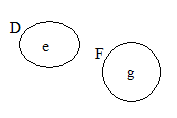3. De ^ Fg = ____ 4. De v Fg = ____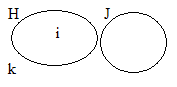5. Hi ^ Jk = ____ 6. Hi v Jk = ____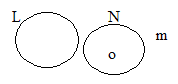7. Lm ^ No = ____ 8. Lm v No = ____9. Ab ^ Cd = ____ 10. Ab v Cd = ____